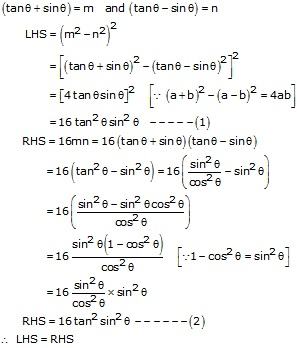## RS Aggarwal Solutions Class 10 Chapter 8 Trigonometric Identities Ex 8b

These Solutions are part of RS Aggarwal Solutions Class 10. Here we have given RS Aggarwal Solutions Class 10 Chapter 8 Trigonometric Identities Ex 8b.

Other Exercises

Question 1.
Solution:Question 2.
Solution:Question 3.
Solution:Question 4.
Solution:Question 5.
Solution:Question 6.
Solution:Question 7.
Solution:Question 8.
Solution:Question 9.
Solution:Question 10.
Solution:Question 11.
Solution:Question 12.
Solution:Question 13.
Solution:Question 14.
Solution:Question 15.
Solution:We hope the RS Aggarwal Solutions Class 10 Chapter 8 Trigonometric Identities Ex 8b help you. If you have any query regarding RS Aggarwal Solutions Class 10 Chapter 8 Trigonometric Identities Ex 8b, drop a comment below and we will get back to you at the earliest.